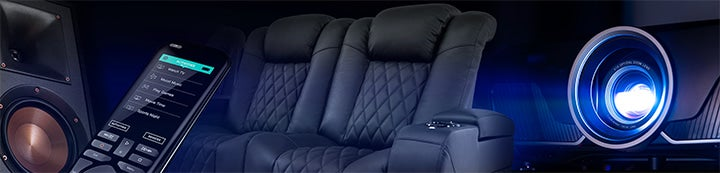1 - 5 of 5 Posts

#### Toscano

·
##### Registered
Joined
·
21 Posts
Discussion Starter · ·
Hi to all, thanks to the hornresp experts (lilmike, soho54 etc) there is now a half-finished horn waiting in the workshop.
Presently, this is a 580 liters enclosure for a TH or OD. Now I am undecided if I should rather build one 580 l / 380 cm horn or a double horn with 2 x 290 liters / 380 cm.
I have compared the single and double horns, and surprisingly, the SPL is not very different between the two. putting 2 drivers in the same large horn doesnt improve the response, apparently. I have also simed one or two OD horns with the same lenght and volume ( and huge Vrc = living-room), but the SPL is lower and the phase response is much worse. I would use the 2 15NDL76 free to air and as offset driver on each side, has this trick been tried and if not, why ? So the internal dimensions of 41 x 75 x 190 cm are fixed ( for the prototype) and I would build 2 of them of course ! The setup will be combined with a sealed speaker from 150 Hz upwards. Thanks for your advice -- Marc
Since the files dont upload, I paste them ( they are for the double horn).
ID=39.00
Ang=1.0 x Pi
Eg=2
Rg=8.00
Fta=1.10
S1=400.00
S2=500.00
Par=60.00
F12=0.00
S2=500.00
S3=1200.00
Par=260.00
F23=0.00
S3=1200.00
S4=1350.00
Par=60.00
F34=0.00
S4=0.00
S5=0.00
L45=0.00
F45=0.00
Sd=855.00
Bl=22.50
Cms=1.87E-04
Rms=5.11
Mmd=84.57
Le=1.50
Re=5.30
TH=1
Vrc=0.00
Lrc=300.00
Ap=0.00
Lpt=0.00
Vtc=0.00
Atc=0.00
Pmax=100
Xmax=8.0
Path=0.0
Fr1=0.00
Fr2=0.00
Fr3=0.00
Fr4=0.00
Tal1=100
Tal2=100
Tal3=100
Tal4=100
Comment=TH.15NDL76

ID=39.00
Ang=1.0 x Pi
Eg=2.00
Rg=8.00
Fta=1.31
S1=400.00
S2=400.00
Par=30.00
F12=0.00
S2=400.00
S3=400.00
Par=30.00
F23=0.00
S3=400.00
S4=1350.00
Par=320.00
F34=0.00
S4=0.00
S5=0.00
L45=0.00
F45=0.00
Sd=855.00
Bl=22.50
Cms=1.87E-04
Rms=5.11
Mmd=84.57
Le=1.50
Re=5.30
OD=1
Vrc=0.00
Lrc=0.00
Ap1=0.00
Lp=0.00
Vtc=0.00
Atc=0.00
Pmax=100
Xmax=8.0
Path=0.0
Fr1=0.00
Fr2=0.00
Fr3=0.00
Fr4=0.00
Tal1=100
Tal2=100
Tal3=100
Tal4=100
Comment=OD.15NDL76

#### LTD02

·
##### Registered
Joined
·
21,345 Posts
two "half horns" located side-by-side should model (and perform) the same as one large horn with two drivers. placement flexibility favors two "half horns" of course.

#### Toscano

·
##### Registered
Joined
·
21 Posts
Discussion Starter · ·
Prototype with options

two "half horns" located side-by-side should model (and perform) the same as one large horn with two drivers. placement flexibility favors two "half horns" of course.
Thanks LTD02 for your quick reply. We are going to glue the enclosure for a double horn this week. I dont want to cut the boards and make 2 separate horns, but rather build two double horns.
Do you think that 2 single horns with a mouth of 1300 cm2 would be enough for a 9 x 10 m room ? I have two sealed push-pull subwoofers with 18" drivers to make a comparison before making the final installation.
The TH produces better results than an OD in hornresp - what is the argument for an OD then ? For aesthetical reasons I wanted to mount the drivers outside the box - it's really not sexy to watch. But since the 15NDL76 has no real bass extension, this would be pointless. Since it is a OSB prototype, I will play with the drivers ( 1 or 2 per side) and use some 15FW76 as an alternative. If I manage to use the measurement set correctly I might post some results. Thank you again ! Marc

#### LTD02

·
##### Registered
Joined
·
21,345 Posts
depends what you are trying to do. the offset driver may give you a little more clean and extended top end. the tapped horn will give you a little more extension and output on the bottom end.

#### LTD02

·
##### Registered
Joined
·
21,345 Posts
while much larger than what you posted, the smoother top end is relatively evident. (that's a 2pi space model btw)

1 - 5 of 5 Posts